×

Search anything:

# Selection sort in C

#### C Programming Algorithms Sorting AlgorithmsIndian Technical Authorship Contest starts on 1st July 2023. Stay tuned.

In this article at OpenGenus, we have explained selection sort and implemented a program on the same in C Programming Language.

• What is Selection sort?
• How selection sort works
• Approach to write the program
• Implementation
• Output
• Time complexity
• Drawbacks of selection sort

# What is Selection sort?

1. Selection sort is one of the most simple and easy sorting algorithms.
2. It works by first finding the smallest element and swapping it with the first element in the array.
3. Then the size of the array is reduced by 1 and the for the rest of the unsorted array step 2 is repeated.
4. Step 3 is repeated until the whole array is sorted.

# How selection sort works

• For example if we have the given array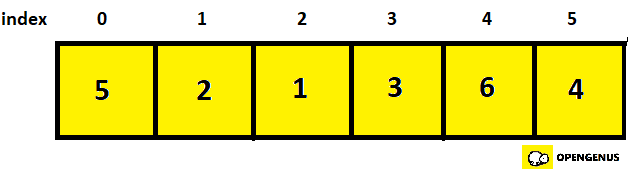• First we select the element at 0th index and compare it with other elements in the array.
• If an element smaller than the element at 0th index is found, then both of these elements are swapped. In the given example, element at 0th index is 5 and the smallest element of the array is 1, at 2nd index, so we swap these two.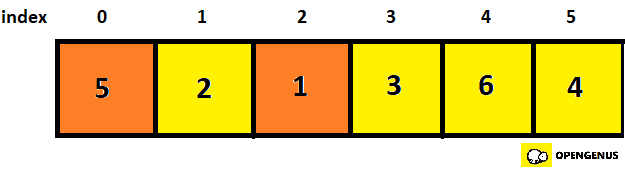• Orange here represents that elements that are to be swapped.
• Now element 1(element at 0th index) is sorted, so we reduce the array size by 1.
• Now element at 1st index is compared with other elements of the array.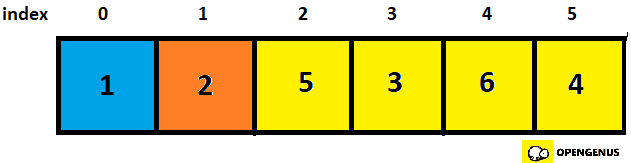• Here blue represents that, this part of the array is sorted andrest is the unsorted part.
• It is found that element at 1st index, that is 2 is the smallest element among the unsorted part of the array so it is not swapped with any other element and array size is again reduced.
• Now we compare the element at 2nd index with the rest of the unsorted array and it is found that element at 3rd index, that is 3 is smaller than element at 2nd index, that is 5.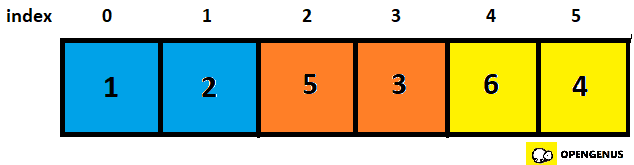• The elements in the orange box(that is the elements 5 and 3) are now swapped.
• Now elements at 3rd index is compared with rest of the unsorted part of the array.
• It is found that element at 5th index is the next smallest element in the unsorted part.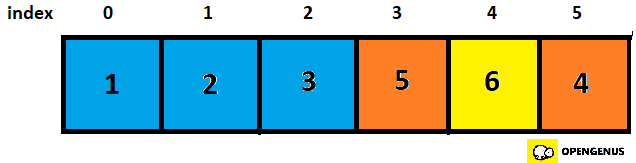• So these two elements are swapped.
• Now next, element at 4th index is compared with the elements in the rest of the array and since only one element is left, elements at index 4 and 5 are compared.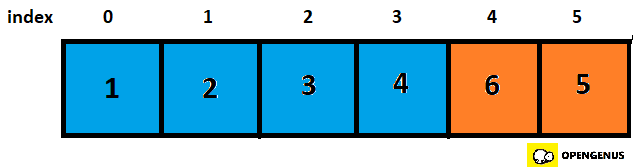• Since element at index 5 is less than element at index 4, they are swapped.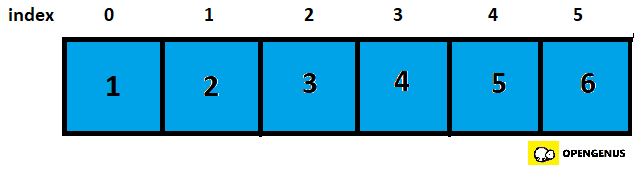• We have gone through the entire array, and now it is sorted.

# Approach to write the program

Following is the approach to solve the problem:

• First we enter the main function.
• Here we declare the array and store the size of the array in variable n.
• Then we call the function selectionSort with the paraments being arr(the name of the array) and n(size of array).
• Now control goes to the function selectionSort.
• Here we run two loops. Outer one will run from 0 to less than n-1 and the inner one will run from i+1 to less than n and is used to find the smallest element in the array which is stored in variable min, then the function swap is called with the parameters being the address of element at min and j as it is a call by reference program.
• Now the two elements are swapped inside the function swap using a third variable.
If element at j is equal to element at min, then no swapping occurs.
• This continues throughout the length of the array until control reaches the end of the array.
• Once it reaches the end of the array, it means that the elements are now sorted.
• Now the sorted array is stored in a new array in a function print and the new array is printed when the function print is called in the main function.

# Implementation

Following is the implemention of the code in C programming language:

``````#include <stdio.h>
void swap(int *p, int *q)
{
int temp = *p;
*p = *q;
*q = temp;
}

void selectionSort(int arr[], int n)
{
int i, j, min;
for (i = 0; i < n-1; i++)
{
min= i;
for (j = i+1; j < n; j++)
if (arr[j] < arr[min])
min= j;
if(min!= i)
swap(&arr[min], &arr[i]);
}
}
void print(int arr[], int size)
{
int i;
for (i=0; i < size; i++)
printf("%d ", arr[i]);
printf("\n");
}
int main()
{
int arr[] = {5,2,1,3,6,4};
int n = sizeof(arr)/sizeof(arr);
selectionSort(arr, n);
printf("Sorted array: \n");
print(arr, n);
return 0;
}
``````

# Output

Run the code as follows:

gcc code.c
./a.out

``````Following is the output of the program:
Sorted array:
1 2 3 4 5 6
``````

# Time complexity

• Since there are two nested loops, each one's time complexity being O(N), the overall time complexity is O(N)* O(N)= O(N^2)

# Drawbacks of selection sort

• Having a time complexity of O(N^2) in both best and worst case makes it unsuitable for large datasets/arrays.
• It cannot take the advantage of array being fully/ partially sorted as it will always check the entire array.